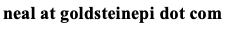# Neal D. Goldstein, PhD, MBI

May 31, 2018

### Assessing the data entry error rate for quality assurance

Many epidemiologists spend a lot of time working with existing data. Not infrequently, these data are derived via an abstraction from other (primary) sources. An example of this is the clinical epidemiologist working with medical data abstracted from the electronic health record. One question that naturally arises is how well do these observed data capture the true data, assuming the other data source - in this case the EHR - is the gold standard. There are a whole host of measurement error and misclassification techniques that can be applied to your sampled data; in this simplistic scenario we just want an idea of the overall error rate (percent that are incorrect). Before we can account for the error (explain, adjust, etc.) we need to understand its presence. To do this we can create an audit dataset that is then used for comparison against the gold standard to compute the error rate.Two questions naturally arise:

1. How do I sample from an existing dataset to create an audit dataset?
2. How many data points do I need?

Let's tackle the second question first. This can be thought of as a one-sample test of proportions (the error rate). We want to see if audited data error rate (p) is outside of a threshold of acceptability (p0). Our null hypothesis is the audited error rate is equivalent to the threshold: p = p0. Our alternative hypothesis is that the audited error rate is less than a threshold for acceptability: p < p0. Therefore this is a one-sided test. Although we hope not to see it, we can also detected if the audited error rate is greater than a threshold for acceptability: p > p0.

Now for some assumptions. We'll accept a false positive rate of 5% (alpha=0.05) and a false negative rate of 20% (beta=0.20). Our threshold for acceptability is 10% error rate, we hope to see the calculated error below this value. To specify the effect size we can imagine a window around this threshold, and whether the true error rate will fall below of this window. The more certain we want to be the actual error is not in this window (by shrinking the window), the larger the sample. For example, if we believe the audited error rate will be 9% and our threshold is 10%, this will require a much larger sample to detect compared to an audited error rate of 5% and a threshold of 10%. The corollary to this - to reject the null hypothesis and conclude the audited error rate is below a threshold - will depend on how sure we want to be of the actual error rate. For this exercise, I assume p = 0.07 and p0 = 0.10.

Plugging these numbers into a sample size calculator tells us we need a sample of 557 data points. Users of R can calculate this by plugging in the following code:

```p=0.07
p0=0.1
alpha=0.05
beta=0.20
n=p0*(1-p0)*((qnorm(1-alpha)+qnorm(1-beta)*sqrt(p*(1-p)/p0/(1-p0)))/(p-p0))^2
ceiling(n) # 557
```

Now, to return to the first question, this can be a simple random sample from the data. Suppose you have a dataset of 1000 observations with 50 variables. Does the number 557 suggest you check one variable for 557 people, or do you check all 50 variables for 12 people (rounding up)? This comes down to the independence assumption. The sample size calculation stipulates you need 557 data points, assuming they are independent from one another. Is there reason to suspect that one observations versus another is more likely to have data entry errors? Or if there were different people abstracting the data, would that affect the data entry? These are important questions to consider as they may affect the error. If there is some correlation suspected, the net effect is loss of data. A straightforward solution is to bump up the sample size to account for the correlated data.

In practice, it is probably desirable to sample a range of observations and variables to ensure as complete coverage as possible to fulfill the calculated number of data points. Then the error rate, p, can be calculated during the audit. With p obtained from the data, one can then calculate a z-statistic and p-value to conclude the hypothesis test. R code as follows:

```z=(p-p0)/sqrt(p0*(1-p0)/n)
p_value = 2*pnorm(-abs(z))
```